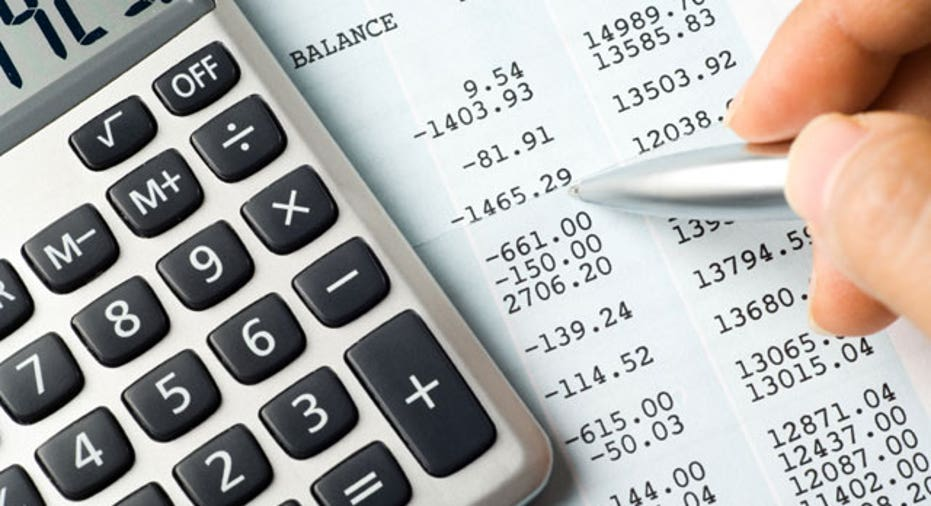# How to Project Sales for Tax PurposesIn last weeks’ article, I discussed how to calculate the cost of creating your product, but it’s just as important for small business owners to know how to project your sales.

We begin first with a break even analysis. Working backwards through the formula for cost accounting will show how many cheesecakes you must create to break even--or if you have a service business, how many worker hours are required to break even.

Example:  Using the cheesecake factory idea, fixed costs total \$1,700.00 per month. Work backward through the equation to determine your break-even point - zero.  In order to reach a profit of zero, how many cakes must you sell?

Manufacturing (Cheesecake)                                                            Break Even Point

\$10.00   (sale price)                                                                             400 Cakes

5.00  (ingredients, direct labor)

.75  (packaging/stickers)

\$5.75  total variable costs

\$4.25  gross profit per cake x 400 =                                                                                                                                                                          \$1,700.00  Gross profit

-1,700.00  Fixed costs

\$     -0-       Profit

If profit is zero and fixed costs are \$1,700.00, then gross profit must be \$1,700.00.  Divide the gross profit of \$1,700.00 by \$4.25 (the gross profit per cake) and you get 400. If you sell less than 400 cakes in a month, you will not achieve sufficient gross profit to cover your fixed costs.

Multiply the quantity of 400 by the selling price of each cake - \$10.00.  Also, multiply the variable cost per cake by the quantity of 400.  Let us examine the full equation:

Manufacturing (Cheesecake)                                          Break even Point

Quantity = 1                                                                         Quantity = 400

\$10.00           Sales                                                                  \$4,000.00

5.00          Ingredients, direct labor                             2,000.00

.75          Packaging / stickers                                          300.00

\$5.75           Total variable costs                                      \$2,300.00

\$4.25           Gross profit                                                     \$1,700.00  Gross profit

- \$1,700.00  Fixed costs

\$      -0-      Profit

You want to do better than break even, so it’s time to project future profits.  What is your goal?  Let’s start with a profit of \$2,550.00.  Using that figure, calculate how many cakes you must sell.  Work backward through the equation again.  Add the \$1,700.00 fixed cost figure to the projected profit of \$2,550.00.  You arrive at \$4,250.00 gross profit.  You already know that your gross profit per cake is \$4.25.  Divide \$4,250.00 by the gross profit per cake to determine the projected quantity -- 4,250.00 ÷ 4.25 = 1,000.  Extend sales and variable costs by multiplying the quantity by the sales price and variable costs per cake.

Quantity = 1                                                                                                     Quantity = 1,000

\$10.00                      Sales                                                                                      \$10,000.00

5.00                    ingredients/direct labor                                                     5,000.00

.75                    packaging/stickers                                                                    750.00

\$5.75                     total variable costs                                                            \$ 5,750.00

\$4.25                     gross profit                                                                           \$ 4,250.00

-\$ 1,700.00 fixed costs

=\$ 2,550.00 profit

Once you have the idea, you can play with the numbers.  Of course, you must take into consideration other factors, such as the capacity of the cheesecake factory.  Perhaps the facilities need to be upgraded in order to produce as many cheesecakes as the projected profit dictates.